Open in App
Not now

# Fundamental Theorem of Calculus

• Last Updated : 30 Jun, 2021

In mathematics, calculus is a branch that helps to understand changes between values that are related to function. It is widely used in the fields of physics, engineering, medicine, economics, biology, space exploration, statistics, pharmacology, and many more. Without calculus, even a house can’t be built.

The history of calculus dates back to ancient Egypt. Historians say that the method used by Egyptians to calculate the volume of a pyramidal frustum was integral calculus. They knew about the basic functions of integral calculus and used them to calculate volumes and area. However, the modern calculus was developed by Isaac Newton and Gottfried Wilhelm Leibniz independently.

There are two branches of calculus:

1. Differential Calculus: In this branch of calculus, we divide things into different pieces and study how they change from one moment to the next. For example, change in velocity with respect to time.
2. Integral Calculus: In this branch of calculus, we join the small pieces together to understand their overall behavior. For example, in determining the length of power cable required to connect the two substations.

In this article, we will be discussing the fundamental theorem of calculus which joins the two branches of calculus, but before that, we first need to understand the area function.

### Area Function

Let us consider a function f(t) continuous in the interval [a, b], as shown in the below image: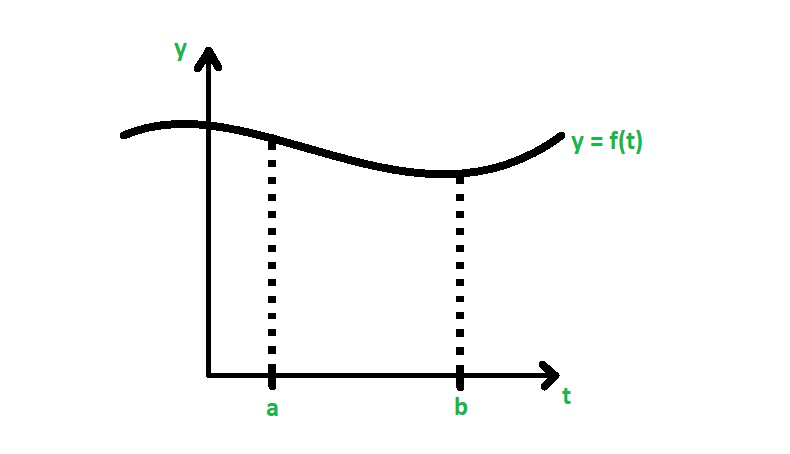Now, let’s mark some point x between a and b on the graph.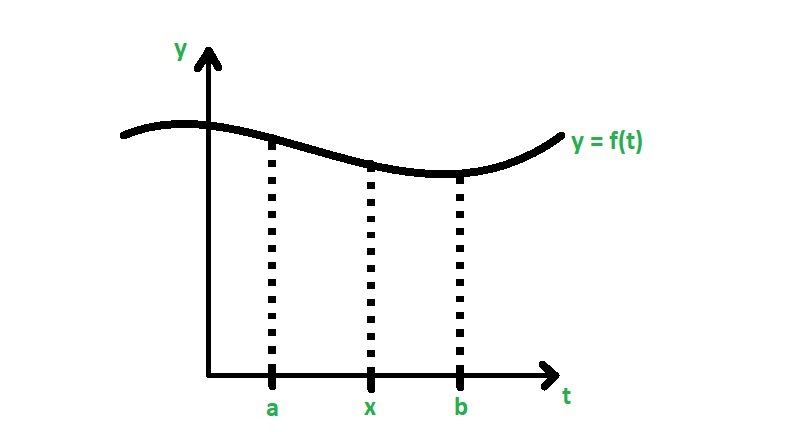Now, we must find the area under the curve y = f(t) between the interval [a, x].

So, the area under the curve between a and x is the definite integral from a to x of f(t) dt, is

A(x) = ∫ax f(t) dt

Here A(x) is known as the area function and it is helpful in finding the fundamental theorem of calculus. Or in other words, A(x), i.e.,  ∫ax f(t) dt is the area of the region bounded by the curve y = f(t) on t-axis with coordinates a and b. If x is a point in between [a, b] interval then ∫ax f(t) dt will represent the area of the shaded region, which is A(x).

Example:

Let’s find out the value of A(x) for function y = 2x between x = 2 and x = 6.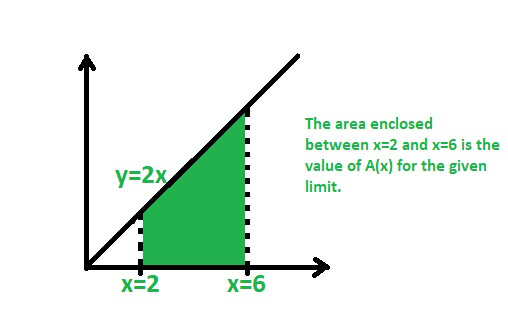A(x) = ∫26 2x dx = [x2]26 = 62 – 22 = 36 – 4 = 32

### The fundamental theorem of calculus

The fundamental theorem of calculus is the powerful theorem in mathematics. It set up a relationship between differentiation and integration. Now, this relationship gives us a method to evaluate definite internal without calculating areas or using Riemann sums. The fundamental theorem is divided into two parts:

1. First fundamental theorem
2. Second fundamental theorem

Now we will discuss each theorem one by one in detail:

### First Fundamental theorem

The first fundamental theorem states that if f(x) is a continuous function on the closed interval [a, b] and the function F(x) is defined by

dF/dx = d/dx(∫ax f(t) dt) = f(x)

Or F'(x) = f(x) over [a, b]

Or in other words, if f is a continuous function on the closed interval [a, b] and the area function is A(x), then

A'(x) = f(x) ∀ x ∈ [a, b]

Proof:

According to the fundamental theorem of calculus, we have

F'(x) = ∫ax f(t) dt = f(x)           …(A)

Now, the equation (A) can be written as

F'(x) = lim h⇢0 (F(x + h) – F(x)/h

= lim h⇢0 1/h [( ∫ax+h f(t) dt – ∫ax f(t) dt )]       …(B)

Thus, equation (B) represents the area under the curve y = f(t) on the

interval [x, x + h]. Now, equation (B) can be written as

F'(x) = lim h⇢0 1/h (∫xx+h f(t) dt         …(C)

Now, according to the mean value theorem of the definite integral,

if there exists a c such that x ≤ c ≤ x + h then

f(c) = 1/h ∫xx+h f(t) dt

Thus,

F'(x) = lim h⇢0 f(c)

Since c is in between x and x + h, therefore c⇢x as h⇢0.

Also, since f(x) is continuous, therefore we have,

lim h⇢0 f(c) = lim c⇢x f(c) = f(x)

Thus,

F'(x) = lim h⇢0 1/h ∫xx+h f(t) dt

= lim h⇢0 f(c)

= f(x)

Hence, proved.

Note:

• Every continuous function f has an antiderivative F(x).
• The fundamental theorem of calculus connects integral calculus and differential calculus.

Finding derivative using the fundamental theorem of calculus:

Let us discuss this concept with the help of an example:

Let’s say we have a function F(x) = ∫21x √(t3) dt and we have to find F'(4).

So, according to the fundamental theorem of calculus, the given equation becomes

F'(x) = √(x3)

Thus, F'(4) = √(43) = 43/2 = (41/2)3 = (√4)3 = 23 = 8.

This is how we solve questions when we have to apply the fundamental theorem of calculus.

Finding a derivative with the fundamental theorem of calculus: x is on both bounds:

Let us discuss this concept with the help of an example:

Suppose there’s a function y = f(t) = sin t/t which is continuous in [x, x2] and we have to find it’s antiderivative. Let’s consider the following diagram :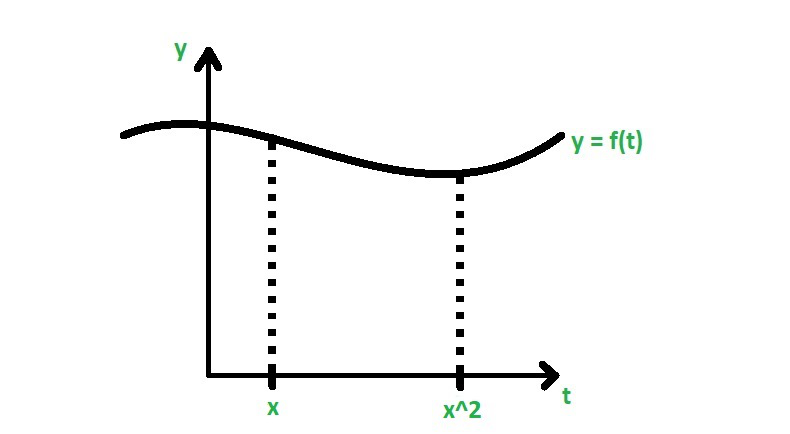According to the fundamental theorem of calculus,…..(1)

Now, mark c on the graph somewhere in between x and x2 on the graph.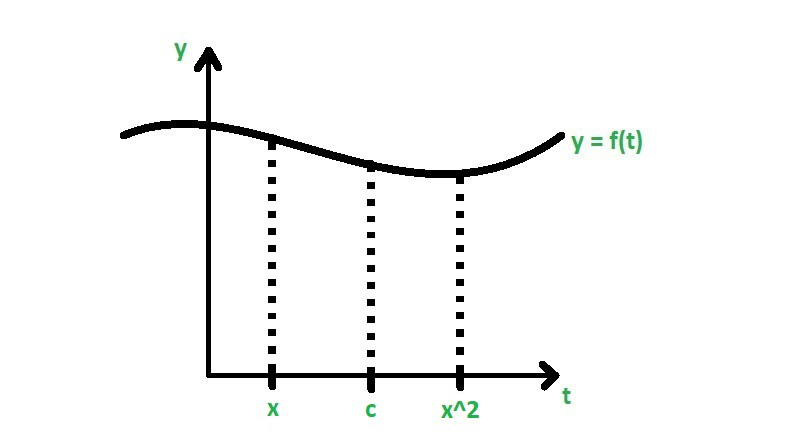Now, equation (1) becomes

dF/dx = d/dx ( ∫xc sin t/t dt ) + d/dx ()

= – d/dx ( ∫cx sin t/t dt ) + d/dx ()

= – sin x/x + (sin x2/x2).2x

= (2 sin x2 – sin x)/x

Thus, we get

F'(x) = (2 sin x2 – sin x)/x

Hence, this is how we find derivatives of the functions where x is on both bounds.

### Second fundamental theorem of calculus

The second fundamental theorem of calculus states that, if f(x) is continuous on the closed interval [a, b] and F(x) is the antiderivative of f(x), then

ab f(x) dx = F(b) – F(a)

The second fundamental theorem is also known as the evaluation theorem.

• This theorem says that ∫ab f(x) dx = the value of the antiderivative F of “f” at the upper limit b – the same antiderivative value at the lower limit a.
• In ab f(x) dx expression, the function f(x) must be well defined and continuous in [a, b] interval.
• It is a very helpful theorem. It provides a method of estimating the definite integral without finding the sum’s limit.
• Here, in estimating the definite integral, the main operation is finding a function whose derivative is the equation to integrated and this process will strengthen the differentiation and integration relationship.

Proof:

Let us consider M = xi, i = 0, 1, …, n is a regular partition of [a, b]

Then

F(b) – F(a) = F(xn) – F(x0)[F(xi) – F(xi – 1)]   …..(1)

Now, As we know that F is the antiderivative of f on the closed interval [a, b],

So using the mean value theorem, we get

i = 0, 1, 2, …,n

Now we find the value of ci in [xi-1, xi]

So,

F(xi) – F(xi – 1) = F'(ci)(xi – xi-1) =f(ci)△x

Now put this value in equation(1), we get

F(b) – F(a) =f(ci)△x

Now taking limit on both sides, i.e., n -> ∞, we get

F(b) – F(a) = limn->f(ci)△x = ∫ba f(x)dx

Hence proved

### Sample Problems

Question 1. Calculate the derivative of the functionat x = 3.

Solution:

Given thatSo, using first FTC, we get

F'(x) == √(x2 + 3x)

Therefore,

F'(3) = √(32 + 3.3) = √(9 + 9) = √(2.9) = 3√2.

Question 2. Calculate the derivative of the functiondt at x = π/2.

Solution:

Given thatSo, using first FTC, we get

F'(x) == f(x)

Hence,

F'(x) = √(sin x + cos x)

Therefore,

F'(π/2) = √(sin π/2 + cos π/2) = √(1 + 0) = 1.

Question 3. Find the derivative of.

Solution:

Given thatSo, let x2 = u.

Now, consider a new function,

G(u) = ∫3u dt/t2

⇒ G'(u) = 1/u2

Since, F(x) = G(x2)

⇒ F'(x) = G'(x2).2x

⇒ F'(x) = (1/x4).2x

⇒ F'(x) = 2/x3.

Question 4. Find the derivative of the.

Solution:

Given thatSo, let x2 = u.

Now, consider a new function,

G(u) = ∫0u √(1 + t3) dt

Using first FTC, we have

G'(u) = √(1 + u3)

As F(x) = G(x2)

⇒ F'(x) = G'(x2).2x

⇒ F'(x) = 2x √(1 + (x2)3)

⇒ F'(x) = 2x √(1 + x6).

Question 5. Find the derivative of the function.

Solution:

Given thatSo, let x2 = u.

Now, considering new function,

G(u) =  ∫1u (t2 + t) dt.

Using first FTC, we get

G'(u) = u2 + u

Since, F(x) = G(x2)

⇒ F'(x) = G'(x2).2x

⇒ F'(x) = (x4 + x).2x

⇒ F'(x) = 2x5 + 2x2.

Question 6. Evaluate the integral.

Solution:

Given thatNow we find the anti-derivative of t

∫t dt = t2 + C

Now using the second FTC, we get

ab f(x) dx = F(b) – F(a)= F(x2) – F(x)= ((t2)2) – (t2)= (t4) – (t2)= t2((t2) – 1)

Question 7. Evaluate the integral.

Solution:

Given that I =Now using the second FTC, we get

ab f(x) dx = F(b) – F(a)= F(2) – F(1)

Now we find the anti-derivative of x

∫x dx = x2 + C= (22) – 1

= 4 – 1

= 3

Question 8. Evaluate the integral ∫02 (x2 – x) dx.

Solution:

Given I = ∫02 (x2 – x) dx

Using second FTC, we get

ab f(x) dx = F(b) – F(a)

02 (x2 – x) dx = F(2) – F(0)

Now we find the anti-derivative of x

02 (x2 – x) dx = x3/3 – x2/2

So,

= [x3/3 – x2/2]02

= [8/3 – 4/2]

= [8/3 – 2]

= [8/3 – 6/3]

= 2/3.

Question 9. Evaluate the integral  F(x) = ∫0x et + e-t dt.

Solution:

Given that F(x) = ∫0x et + e-t dt

Using the first FTC, we get

F'(x) = ex + e-x.

Question 10. Find the integral of.

Solution:

Given I =so we can also write as

I =Now apply integration by parts, we get

u = t, dv = d(e-t)

So, du = 1, v = e-t

So, the integral is

I = –=== -e-ln2(ln2 + 1) + e0 .1

= 1/2(ln e – ln 2)

= 1/2ln e/2

My Personal Notes arrow_drop_up
Related Articles